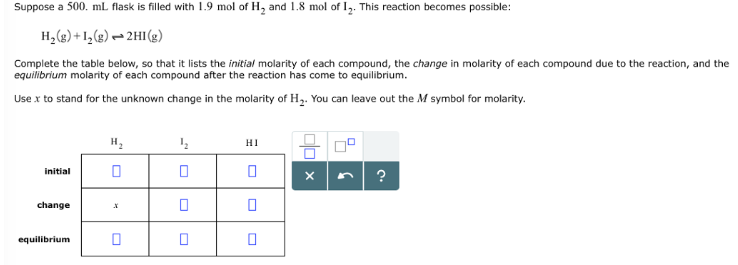# Problem: Suppose a 500 mL flask is filled with 1.9 mol of H2 and 1.8 mol of I2. This reaction becomes possible: H2(g) + I2(g) ⇌ 2HI(g) Complete the table below, so that it lists the initial molarity of each compound, the change in molarity of each compound due to the reaction, and the equilibrium molarity of each compound after the reaction has come to equilibrium.Use x to stand for the unknown change in the molarity of H2. You can leave out the M symbol for molarity.

###### FREE Expert Solution
99% (300 ratings)###### Problem Details

Suppose a 500 mL flask is filled with 1.9 mol of H2 and 1.8 mol of I2. This reaction becomes possible:

H2(g) + I2(g) ⇌ 2HI(g)

Complete the table below, so that it lists the initial molarity of each compound, the change in molarity of each compound due to the reaction, and the equilibrium molarity of each compound after the reaction has come to equilibrium.

Use x to stand for the unknown change in the molarity of H2. You can leave out the M symbol for molarity.